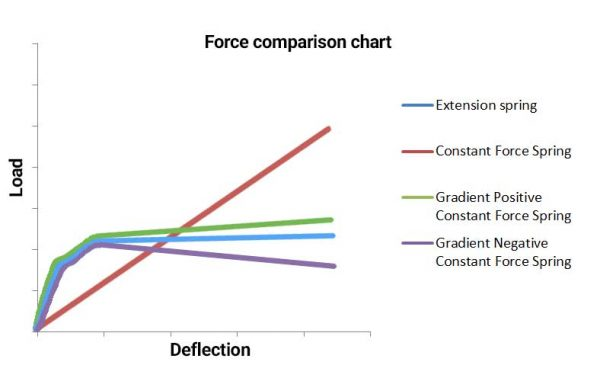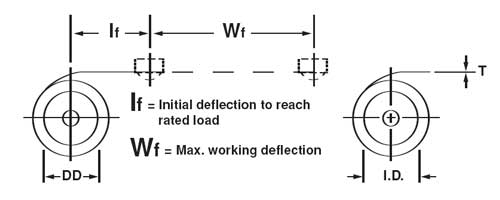# Constant force spring: properties and design equations

Constant force springs differ from traditional helically-wound springs. They are made from pre-tensioned, high yield strips of metal (often stainless steel) as opposed to wire.

Constant force springs are classified as “extension springs”. When coiled, the metal strip resists uncoiling with a near-constant resistance force. When extended, the internal stress of the metal resists any loading force at a near-constant rate.

Once the spring is extended to a length of 1.25 times its diameter, full load is reached, and a near-constant force is created. Depending on the size of the spring and the load it’s supporting, constant force springs have a fatigue life cycle of between 2,500 cycles to 1,000,000 cycles.

## Properties of constant force springs

Constant force springs don’t exhibit the same results as springs bound by Hooke’s law (that extension force is proportional to the extended length). Therefore the force constant of a spring doesn’t apply as a near-constant force is applied when a specific maximum load is reached (see the graph below).If you are choosing a constant force spring for a certain application, you must first take into account several factors.

Material choice is important, as well as the thickness and width of the metal strip. The most common metal used is stainless steel grade 301, as it makes a consistently high-quality and robust constant force springs. Other materials are available such as high-carbon steel, Inconel, etc. which may be more suitable for springs that need to resist high loading forces.

To choose or manufacture the correct spring, you must know the approximate initial force or load that the springs need to overcome to reach the “constant force” stage (i.e. the flat section of the blue line on the graph above). The higher the initial load, the greater the diameter spring you will need.

Also, the spring end detail needs to be designed to hold or attach to the component that will coil and uncoil it.

## Mounting a constant force spring

To decide upon the dimensions of constant force springs, certain guidelines must be followed.• The constant force spring is initially lightly wrapped around a drum
• The free end is attached to a loading force (like a counterbalance application)
• The diameter of the drum needs to be 10 to 20% higher than the natural strip diameter
• A minimum of 1.5 wraps of the strip should be on the drum at full extension
• For long extensions, the strip should be guided to stop kinking or twisting when recoiling

To calculate the total length of the spring strip, the following equation can be used:

Total length = circumference wrapped on the drum + initial deflection + max working deflection

= (I.D.  x  π x  1.5) + If + Wf

For standard springs that obey Hooke’s law, a simple torsion spring calculator can be used to calculate the spring constant and thus to adjust lengths and loading forces to suit the application. This can be used for any torsional, extension or compression spring that doesn’t reach a near-constant force at a specific load.

As constant force springs do not obey Hooke’s law, proportionality cannot be applied, so the equations below must be used instead:

For N ≤ 10

P=Ebt3/6.5DD2

For N > 10

P=Ebt3/6.5D1(2/Dn-1/D1)

S=Et/Dn

## Where:

E=Modulus of Elasticity (Pa, psi)

D1=outside coil diameter (mm, in)

DD=drum diameter (mm, in)

Dn= natural diameter (mm, in)

b=spring width (mm, in)

t=thickness (mm, in)

N=Number of coil turns

S=stress Mpa (psi)

For typical designs, ratio b/t = 100 and ratio DD/Dn = 1.2.

## Applications of constant force springs

Constant force springs are ideal for applications that require smooth returning, counterbalancing and tensioning or reloading. This video highlights some of the applications that take advantage of these functions.

Constant force springs are commonly used in automotive engineering, aerospace engineering, medical and retail.

Some common applications are:

• Cable retractors
• Electric motors
• Space vehicles
• Gym equipment
• Door closers
• Hose retrievers
• MRI scanners
• X-ray machines
• Point of sale displays
• Toys
• Furniture parts
• Retractable tape measures or dog leads
• Retractable vacuum cleaner cords

## Limitations of constant force springs

External factors can limit the life cycle of constant force springs.

For instance, debris or particulates can clog the system, slowing down or impeding coiling and uncoiling. If the spring mechanism is open to the elements or is in regular contact with corrosive fluids, corrosion can take place, compromising the movement of the spring.

Extreme temperatures may also affect the spring operation. This may be of particular concern in aerospace engineering.

• Constant force springs are commonly used, but how much do you actually know about them?
• Discover what constant force springs are, how they differ from standard springs and what they are used for
• Learn how to use spring force equation to calculate the total length of the spring strip